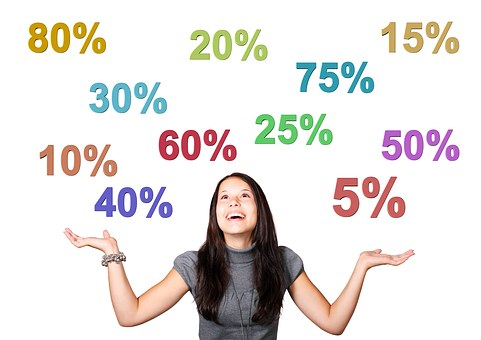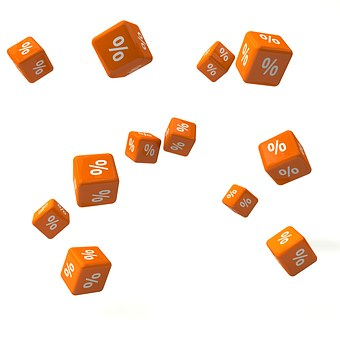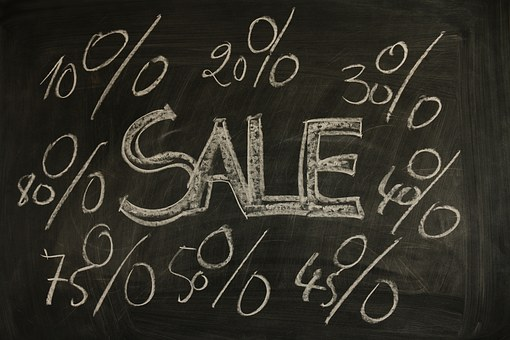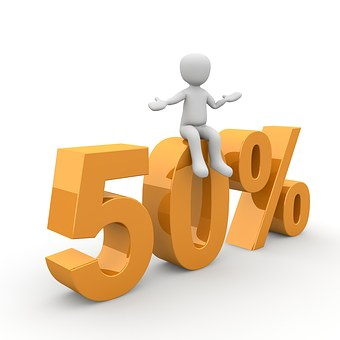# GMAT Percentages: 5 Key Tips for Percent ProblemsPercents are one of the most common math concepts: in fact, you probably use them every day, whether calculating a tip or determining how much a discount really is. It’s not surprising, then, that GMAT percentage questions pop up throughout the quant section. You may see percents tested by themselves, or they might be part of another question type, such as a geometry question.

Unfortunately, GMAT percent problems can be quite tricky even though the concepts they test are fairly simple. But don’t worry! In this guide, I’ll explain exactly what you need to know to master percentage questions on the GMAT and walk you through sample problems using percents.

## What’s Tested in GMAT Percentage Questions?

For many test takers, GMAT percentage questions seem very straightforward and easy. After all, we deal with percentages often in day-to-day life, too calculate taxes, interest rates, tips, and more. However, while GMAT percentages may appear easier than say, standard deviation questions, they can actually be quite tricky. Before we get into some tips for how to solve GMAT percentage questions, let’s talk a bit more about what this question type actually tests.

The word percent can be broken down into “per cent,” which means “through 100.” A percent is a part of 100. The formula for finding a percent is: \$\part/\whole * 100\$.

For the GMAT percent problems, you’ll mainly be concerned with calculating percent change. A percent change asks you to find the difference in percent between a starting and ending number.

Not sure how or what to study? Confused by how to improve your score in the shortest time possible? We've created the only Online GMAT Prep Program that identifies your strengths and weaknesses, customizes a study plan, coaches you through lessons and quizzes, and adapts your study plan as you improve.

We believe PrepScholar GMAT is the best GMAT prep program available, especially if you find it hard to organize your study schedule and don't want to spend a ton of money on the other companies' one-size-fits-all study plans.The formula for finding percent change is:

\$\$\percent \change = (\amount \of \change)/(\starting \amount) * 100\$\$

Let’s look at that in action.

If the price of an item changes from 10 dollars to 15 dollars and we want to find the percent increase, we would plug values into our equation. The amount of change from 10 to 15 is 5 (15 – 10 = 5) and the starting amount is 10. Thus our equation would be:

\$\$\percent \change = (5 / 10) * 100\$\$

\$\$\percent \change = 50%\$\$

Percentages will often pop up in geometry and arithmetic questions. For geometry questions, you may be asked to determine by what percentage the area of a square increases if the length of its sides is increased by 20%. Or, you may be asked to determine how a decrease in the area of a triangle corresponds to its sides.

You’ll see all kinds of applications in arithmetic questions. You may be asked to calculate the percent change of a savings account, or the profits of a company. You may be asked to calculate percent change of the output of cookies from a popular bakery. Whatever you’re asked to calculate, make sure that you follow the tips suggested in the following section.## 5 Key Tips for Solving GMAT Percentages

As I mentioned before, GMAT percent problems can be deceptively tricky. In this section, I’ll outline the most important tips to keep in mind as you’re attacking GMAT percentages.

### #1: Language Matters

One of the biggest challenges with percentage questions is tricky wording. You need to read percentage question doubly carefully to make sure that you’re answering the correct question.

Consider the following example:

If you’re purchasing a bicycle that costs 500 dollars, and its price suddenly doubles, the cost of the bicycle is now 1,000 dollars. 1,000 dollars represents an increase of 100% from the original price of the bike, but 200% of the original cost of the bike.

See how the wording there is tricky? Depending on whether you’re searching for the percent change (increase) or trying to determine the proportion of the original cost of the bike, you’ll be looking for two different numbers. It can be easy to fly through a question like this and accidentally pick the wrong right number, if you’re not reading carefully.

### #2: Mind the Increase-Decrease Trap (or the Decrease-Increase Trap)

Consider the following example:

The price of watermelon increased by 30%, and then decreased by 30%. The final price is what percent of the original price?

This type of question is very common on the GMAT and is designed to trick you. Inexperienced test-takers may see the increase of 30% and the decrease of 30% and think that 100% will be the correct answer because, in theory, the price of the good has increased and decreased by the same amount.

Not so.

When you go up by a percent and then down by the same percent, you don’t end up with the amount that you started with. For instance, if you are increasing the price of a watermelon by 30%, you’ll use that new price as the starting value to solve the next part of the question. Having different starting values means that you’ll have different answers. In order to find the actual answer, you’ll have to multiply the two multipliers. I’ll explain what that means more in the next tip.### #3: In a Series of Percentage Changes, Multiply the Multipliers

In order to find the percent change in a series of percentage changes, you must multiply the multipliers. Let’s consider the example in the previous question.

The price of the watermelon initially increased by 30%. We can represent that increase as 1.3. That’s the multiplier we’ll use for the increase.

The price of the watermelon then fell by 30%. We can represent that decrease as 0.7.

In order to find the total change, we need to multiply the multipliers.

\$\$(1.3)(0.7) = 0.91\$\$

The watermelon’s final price is 91% of the initial price, which means it actually decreased by 9 percent.

Want to improve your GMAT score by 60 points?

We have the industry's leading GMAT prep program. Built by Harvard, MIT, Stanford, and Wharton alumni and GMAT 99th percentile scorers, the program learns your strengths and weaknesses and customizes a curriculum so you get the most effective prep possible.Whenever you have more than one multiplier, multiply the multipliers to find the actual percent change.

### #4: Starting Value Matters

Make sure you know which value is the starting value when you’re trying to determine percent change.

Remember, we calculate percent change using both the amount of change and the starting value. If we have either of these numbers incorrect, the answer will be wrong.

Take a look at this example:

The price of a good is now 400 dollars, having decreased from 500 dollars.

In this example, the starting value is 500 dollars. The language in this question is slightly confusing and 500 dollars appears second in the statement. Only by reading carefully can you actually find out which value is the starting value.

If you use the incorrect starting value, you won’t be able to get to the right answer. Make sure you’re using the correct starting value as you’re working through a question.

### #5: Know Your Multipliers

There are three main kinds of multipliers that you’ll see on the GMAT. Knowing which one to use when is key to finding the correct value on percent problems.

#### X% of a number

You can find X% of a number by multiplying the number by the percent expressed as a decimal. What does that mean?

Say you’re trying to understand what 35% of 500 is. You’ll multiply 500 by 35 percent to find the correct answer. You would express this in equation form as:

\$\$(0.35)(500) = x\$\$

We can say that the formula for finding X% of a number is:

(percent as a decimal)(original value)

#### X% increase

An increase multiplier is expressed as:

multiplier = 1 + (percent as a decimal)

If you were to use an increase multiplier to find the increase in a value, you would multiply the increase multiplier by the original value. Let’s look at what that means.

Say that the money in your savings account increases 15% over the year from its original value of \$500.

To find the percent increase you would use the following equation:

Want to Identify YOUR GMAT Strengths and Weaknesses?

Our proprietary GMAT Diagnostic Assessment creates a customized study plan for you that takes you from registration all the way to test day! It is included with every account and proven to significantly maximize your score.

Get your personalized assessment as part of your 5 day risk-free trial now:\$\$(1.15)(500) = X\$\$

I used the number 1.15 because to find the multiplier of a percent increase problem, I add 1 to the percent expressed as a decimal, e.g. (1 + .15 = 1.15).

#### X% decrease

A decrease multiplier is expressed as:

multiplier = 1 – (percent as a decimal)

If you were to use a decrease multiplier to find the decrease in a value, you would multiply the decrease multiplier by the original value.

Say the money in your savings account decreased 15% over the year from its original value of \$500.

To find the percent decrease, you would use the following equation:

\$\$(0.85)(500) = x\$\$

I used 0.85 because, to find a decrease multiplier, I subtract the percent decrease from 1. (1 – .15 = 0.85)

These multipliers often trip up test takers. Many test takers struggle with using the correct formulas to find percent increase and percent decrease. Make sure you remember to add or subtract the percent increase or decrease from 1 in order to get the correct multiplier.## GMAT Percent Problems Examples

Check out these sample GMAT percent problems to see how I solve a variety of GMAT percent problems using the tips I described above.

### Problem Solving GMAT Percentages Sample Question

Positive integer y is 50 percent of 50 percent of positive integer x, and y percent of x equals 100. What is the value of x?

1. 50
2. 100
3. 200
4. 1,000
5. 2,000

Because y is a positive integer, we can express y percent as y/100. Knowing that, let’s express the information in the question as equations.

We know that x is 50 percent of 50 percent of x, which is a positive integer. We can express that equation as:

\$y = (0.50)(0.50x)\$

We also know that y percent of x = 100. Remember, we can express y percent as y/100, since we know that y is a positive number. We can always express a percent as the value/100 when the value is a positive integer.

We can express the second equation as

\$y/100x = 100\$

Now, let’s simplify that first equation.

\$y = (0.50)(0.50x)\$

\$y = 0.25x\$

Now, let’s simplify the second equation by multiplying each side by 100.

\$yx = 10,000\$

Now we can plug our simplified value of y (y = 0.25x) into the second equation in order to isolate x.

\$(0.25x)(x) = 10,000\$

or

\$0.25x^2 = 10,000\$

Let’s continue to isolate x by dividing each side by 0.25

\$x^2 = 40,000\$

Now let’s find the square root of each side to find the value of x.

\$x = 200\$

The correct answer is C.### Data Sufficiency GMAT Percentages Sample Question

Of the companies surveyed about the skills they required in prospective employees, 20 percent required both computer skills and writing skills. What percent of the companies surveyed required neither computer skills nor writing skills?

1. Of those companies surveyed that required computer skills, half required writing skills.
2. 45 percent of the companies surveyed required writing skills but not computer skills.
1. Statement (1) ALONE is sufficient, but statement (2) alone is not sufficient
2. Statement (2) ALONE is sufficient, but statement (1) alone is not sufficient
3. Both statements TOGETHER are sufficient, but NEITHER statement ALONE is sufficient
4. EACH statement ALONE is sufficient
5. Statements (1) and (2) TOGETHER are NOT sufficient

With data sufficiency questions, we always want to evaluate each statement individually first. We’re trying to determine the percent of companies that required neither computer nor writing skills.

From the information in the questions, we can say that the companies surveyed could be placed into one of the four following categories:

1. Requiring computer skills and requiring writing skills
2. Requiring computer skills but not requiring writing skills
3. Not requiring computer skills but requiring writing skills
4. Not requiring computer or writing skills

It’s given that 20 percent of the surveyed companies fell into category 1. It’s necessary to determine what percent of the survey companies fell into category 4.

Let’s look at statement 1. This helps identify the percentage in category 2. Since ½ the companies requires computer skills also required writing skills, then the other ½ of the companies that required computer skills did not require writing skills. However, this information only establishes that 20 percent required computer skills, but not writing skills. Statement 1 is not sufficient.

Let’s look at statement 2. While this category establishes category 3, that is that, 45 percent required writing skills but not computer skills, no further information is available. Statement 2 is not sufficient.

Now let’s look at them both together.

Taking (1) and (2) together, the first three categories add up to 85 percent (20 + 20 + 45 = 85). Therefore, category 4 would be equal to 100 – 85 = 15 percent of the surveyed companies required neither skill.

The correct answer is C.

## What’s Next?

Looking for more in-depth GMAT quant guides? We’ve got you covered. Our in-depth guides to GMAT geometry, probability, and rate problems offer comprehensive tips and tricks for each of these GMAT content areas. You’ll learn about the types of questions you’ll see on the GMAT, as well as the important formulas and strategies you need to know to succeed.

Our guides to GMAT problem solving and GMAT data sufficiency examine the two GMAT quant question types in greater detail. Each guide focuses on the content areas covered by the question type and offers specific strategies for succeeding at each. It’s important to be equally comfortable solving data sufficiency and problem-solving questions. Use our guides to help you on the path to test day.

If you’re looking for more overall strategy, our guide to the 10 most important tips for beating the GMAT, will help you increase the effectiveness of your GMAT preparedness before and during test day. Learn the most important tips and strategies you should be employing to achieve your goal score.